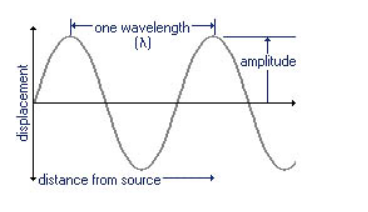# Characteristics of Sound Waves

Sound wave can be described by five characteristics: Frequency, Amplitude, Wavelength, Time-Period, and Velocity.

Frequency: Number of complete waves produced in 1 sec is called frequency of the wave. Since one complete wave is produced by one full vibration of the vibrating body, so we can say that the number of vibrations per sec is called frequency. e.g. if 5 complete waves are produced in 1 sec then the frequency of the waves will be 5 Hz or 5 cycles per second.

Amplitude: If a wave passes through any medium, the particles of the medium get displaced temporarily from their original positions. The maximum displacement of the particles of the medium from their original positions, when a wave passes through the medium is called amplitude of the wave.

Wavelength: Minimum distance in which a sound wave repeats itself is called its wavelength. i.e it is the length of one complete wave. It is denoted by a Greek letter λ (lambda).  We know that in a sound wave, the combined length of a compression and an adjacent rarefaction is called its wavelength. Also, the distance between the centers of two consecutive compressions or two consecutive rarefactions is equal to its wavelength.Time-Period: Time required to produce one complete wave or cycle  is called time-period of the wave. Now, one complete wave is produced by one full vibration of the vibrating body. So, the time taken to complete one vibration is known as time-period.

Velocity: Distance travelled by a wave in 1 sec is called velocity of the wave, represented by the letter v. The S.I unit for measuring the velocity is m/s.

Velocity = Distance travelled / Time taken

Let v = λ / T     (T = time taken by one wave.)

v = f X λ         (This formula is known as wave equation.)

v = velocity of the wave, f = frequency, λ = wavelength

Velocity of a wave = Frequency X Wavelength

Post By : Aarav Yadav 25 Dec, 2017 2199 views Physics